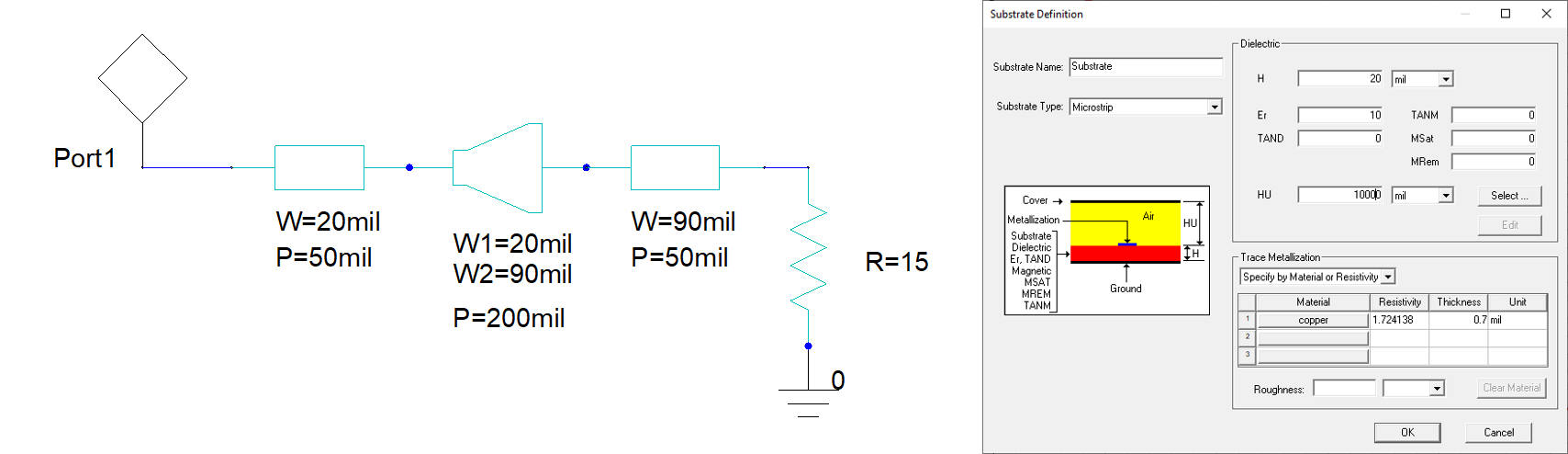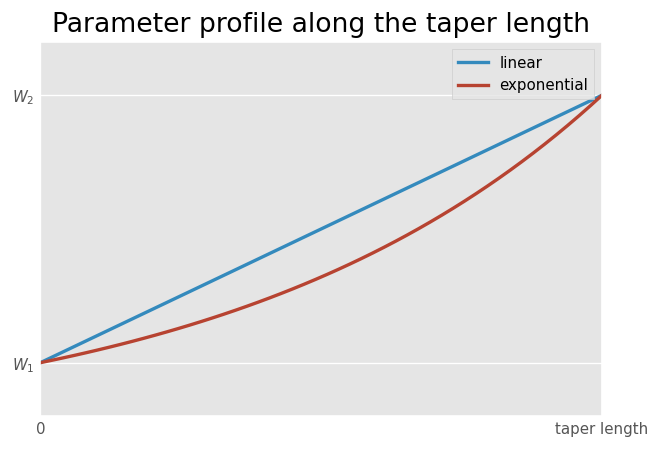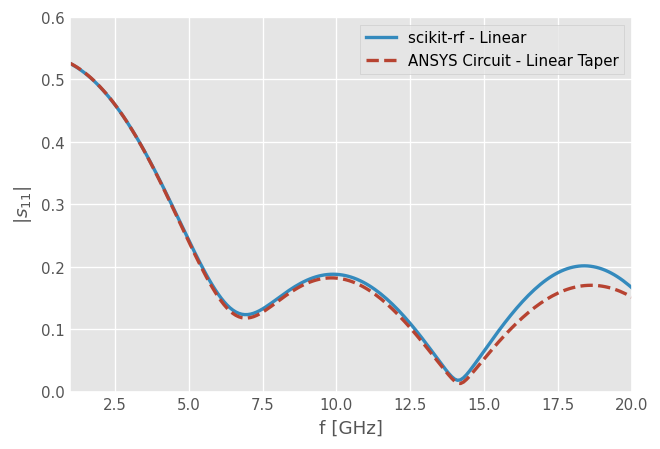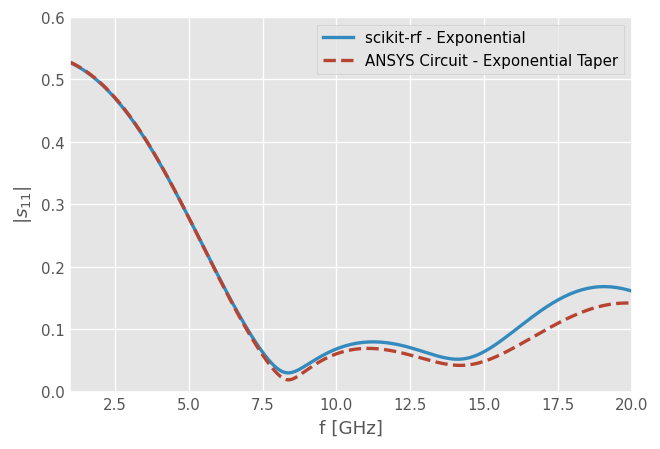# Tapered Transmission Lines¶

A tapered line (or simply a taper), is an impedance transformer that matches an impedance $$Z_1$$ to an impedance $$Z_2$$ using a gradually varying characteristic impedance $$Z(z)$$ along the line.

There is an infinite number of ways one can define a profile $$Z(z)$$ along the transmission line. scikit-rf implements a generic 1D Taper Taper1D, from which the following direct classes are derived: Linear, Exponential and SmoothStep.

:

import skrf as rf
import numpy as np
import matplotlib.pyplot as plt
from skrf.media import MLine

rf.stylely()


## Geometry¶

In this example, we compare a microstrip line which width $$W$$ is tapered and loaded with a 15 Ohm resistor:Before comparing the taper profiles, let’s define the other elements of the circuit, that is the piece of transmission lines and resistor:

:

# Model Parameters
freq = rf.Frequency(1, 20, unit='GHz', npoints=191)
w1 = 20*rf.mil  # conductor width [m]
w2 = 90*rf.mil  # conductor width [m]
h = 20*rf.mil  # dielectric thickness [m]
t = 0.7*rf.mil  # conductor thickness [m]
rho = 1.724138e-8  # Copper resistivity [Ohm.m]
ep_r = 10  # dielectric relative permittivity
rough = 1e-6  # conductor RMS roughtness [m]
taper_length = 200*rf.mil  # [m]

# Media definitions
microstrip_w1 = MLine(freq, w=w1, h=h, t=t, rho=rho, ep_r=ep_r, rough=rough)
microstrip_w2 = MLine(freq, w=w2, h=h, t=t, rho=rho, ep_r=ep_r, rough=rough)

# piece of transmission lines connected to the taper
line1 = microstrip_w1.line(d=50, unit='mil', name='feeder')
line2 = microstrip_w2.line(d=50, unit='mil', name='feeder')

resistor = microstrip_w2.resistor(R=15)


The idea is hence to forge a transmission line of variable characteristic impedance. In this example, the width of the metallization $$W$$ is varied along the transmission line length. Most common profile are summarized in the figure below:

:

fig, ax = plt.subplots()
z = np.linspace(0, taper_length)
ax.plot(z, (w2-w1)/taper_length*z + w1, lw=2, label='linear')
ax.plot(z, w1*np.exp(z/taper_length*(np.log(w2/w1))), lw=2, label='exponential')
ax.set_xticks([0, taper_length])
ax.set_xticklabels(['0', 'taper length'])
ax.set_yticks([w1, w2])
ax.set_yticklabels(['$W_1$', '$W_2$'])
ax.legend()
ax.set_title('Parameter profile along the taper length')

:

Text(0.5, 1.0, 'Parameter profile along the taper length')### Linear Taper¶

:

# create a 2-port Network
taper_linear = rf.taper.Linear(med=MLine, param='w', start=w1, stop=w2,
length=taper_length, n_sections=50,
med_kw={'frequency': freq, 'h': h, 't':t, 'ep_r': ep_r,
'rough': rough, 'rho': rho}).network
print(taper_linear)

# build the full circuit
# equivalent to ntwk = line1 ** taper_linear ** resistor ** line2 ** microstrip_w2.short()
ntwk_linear = rf.cascade_list([line1, taper_linear, line2, resistor, microstrip_w2.short()])

2-Port Network: '',  1.0-20.0 GHz, 191 pts, z0=[47.98835491+0.j 18.86744137+0.j]

:

fig, ax = plt.subplots()
ax.plot(ntwk_linear.frequency.f_scaled, ntwk_linear.s_mag[:,0], lw=2, label='scikit-rf - Linear')

f_ref, s_mag_ref = np.loadtxt('ANSYS_Circuit_taper_linear_s_mag.csv', delimiter=',', skiprows=1, unpack=True)
ax.plot(f_ref, s_mag_ref, label='ANSYS Circuit - Linear Taper', lw=2, ls='--')

ax.set_xlabel('f [GHz]')
ax.set_ylabel('$|s_{11}|$')
ax.set_ylim(0, 0.6)
ax.set_xlim(1, 20)
ax.legend()

:

<matplotlib.legend.Legend at 0x7fe2f1488dc0>### Exponential Taper¶

:

taper_exp = rf.taper.Exponential(med=MLine, param='w', start=w1, stop=w2,
length=taper_length, n_sections=50,
med_kw={'frequency': freq, 'h': h, 't':t, 'ep_r': ep_r,
'rough': rough, 'rho': rho}).network

ntwk_exp = line1 ** taper_exp ** line2 ** resistor ** microstrip_w2.short()

:

fig, ax = plt.subplots()
ax.plot(ntwk_exp.frequency.f_scaled, ntwk_exp.s_mag[:,0], lw=2, label='scikit-rf - Exponential')

f_ref, s_mag_ref = np.loadtxt('ANSYS_Circuit_taper_exponential_s_mag.csv', delimiter=',', skiprows=1, unpack=True)
ax.plot(f_ref, s_mag_ref, label='ANSYS Circuit - Exponential Taper', lw=2, ls='--')

ax.set_xlabel('f [GHz]')
ax.set_ylabel('$|s_{11}|$')
ax.set_ylim(0, 0.6)
ax.set_xlim(1, 20)
ax.legend()

:

<matplotlib.legend.Legend at 0x7fe2f13cd130>[ ]: